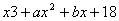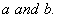# MATHEMATICS — 2004 (Set III — Compartment Outside Delhi)

Except for the following questions, all the remaining questions have been asked in Set I and Set II .

Q. 3. Draw a circle of diameter 7 cm. From a point P, 8 cm away from its centre, construct a pair of tangents to the circle. Measure the lengths of the tangent segments.

Q. 6. Ifis a factor ofand, find.Q. 9. A loan of Rs.16,550 is to be returned in three equal annual instalments. If the rate of interest is 10% per annum, compounded annually, find each annual instalment.

Q. 10. A burner is available for Rs.1,600 cash or for Rs.360 as cash down payment followed by three monthly instalments of Rs.420 each. Find the rate of interest under the instalment plan.

Q. 14. The monthly incomes of A and B are in the ratio of 5 : 4 and their montly expenditures are in the ratio of 7 : 5. If each saves Rs.3,000 per month, find the monthly income of each.

Q. 17. Find the value of k for which the points with coordinates (2, 5), (k,11/2) and (4, 6) are collinear.

Q. 18. The following table gives the marks scored by 80 students in a class-test:

 Marks 0-50 50-100 100-150 150-200 200-250 250-300 Number of Student 8 12 20 25 10 5

Find the mean marks scored by a student in the class-test.

Q. 21. The annual income of Garima (aged 40 years) is Rs.2,80,000 (exclusive of HRA). She contributes Rs.5,000 per month towards provident fund and pays an annual premium of Rs.12,000 towards her LIC policy. She pays Rs.3,000 per month as income tax for the first 11 months. Calculate the income tax payable by her in the last month of the year. For calculating income tax, use the following:

for calculating income tax, use the following:

 (a) Standard Deduction Gross income Deduction (i) Upto Rs.75,000 (ii) From Rs.75,001 to Rs.5,00,000 (iii) More than Rs,5,00,000 40% of gross income Rs.30,000 Rs.20,000 (b) Rates of Taxes Slab Income tax (i) Upto Rs. 50,000 (ii) From Rs. 50,001 to Rs. 60,000 (iii) From Rs. 60,001 to Rs. 1,50,000 (iv) Rs. 1.50,000 and above No tax 10% of the amount exceeding Rs.50,000 Rs.1,000+20% of the amount exceeding Rs.60,000 Rs.19,000+30% of the amount exceeding Rs.1,50,000 (c) Rebate in Tax (i) 20% of the savings subject to a maximum of Rs. 14,000 if the gross income is upto Rs. 1,50,000. (ii)15% of the savings subject to a maximum of Rs. 10,500 if the gross income is from Rs. 1,50,001 to Rs. 5,00,000. (d) Surcharge 10% of the tax payable if theannual income is more than Rs.8,50,000. (e) Rebate for women of less than 65 years Rs. 5,000 over and above other admissible rebates.
Maths 2004 Question Papers Class X
CBSE 2004 Question Papers Class X